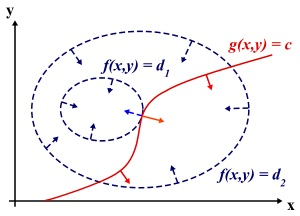# 深度学习：参数正则化参考资料

## Hessian矩阵

• 若$H(m)$是正定矩阵，则M处为局部极大值。

• 若$H(m)$是负定矩阵，则M处为局部极小值。

• 若$H(m)$是不定矩阵，则M处不是极值。

## 拉格朗日乘子和KKT条件

### 一. 拉格朗日乘子法（Lagrange Multiplier) 和KKT条件

1.无约束优化问题，可以写为:

2.有等式约束的优化问题，可以写为:

3.有不等式约束的优化问题，可以写为：

• 拉格朗日乘子法（Lagrange Multiplier): 对于等式约束，我们可以通过一个拉格朗日系数a 把等式约束和目标函数组合成为一个式子 $L(a, x) = f(x) + a*h(x)$, 这里把a和 $h(x)$ 视为向量形式，a是横向量，h(x)为列向量。然后求取最优值，可以通过对 $L(a,x)$ 对各个参数求导取零，联立等式进行求取，这个在高等数学里面有讲，但是没有讲为什么这么做就可以，在后面，将简要介绍其思想。

• KKT条件: 对于含有不等式约束的优化问题，如何求取最优值呢？常用的方法是KKT条件，同样地，把所有的不等式约束、等式约束和目标函数全部写为一个式子 $L(a,b,x)=f(x)+ag(x)+bh(x)$ ，KKT条件是说最优值必须满足以下条件：

1. $L(a, b, x)$ 对 $x$ 求导为零

2. $h(x) =0$

3. $a*g(x) = 0$

求取这三个等式之后就能得到候选最优值。其中第三个式子非常有趣，因为$g(x)<=0$，如果要满足这个等式，必须$a=0$或者$g(x)=0$ . 这是SVM的很多重要性质的来源，如支持向量的概念。

### 二. 为什么拉格朗日乘子法（Lagrange Multiplier) 和KKT条件能够得到最优值？